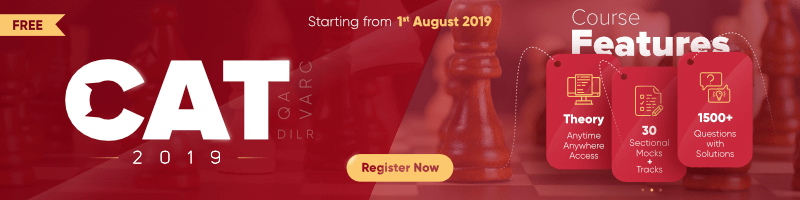# Probability | Set-2

Question 1: If a card is picked up random from the a pack of 52 cards. Find the probability that it is a king or queen.
Solution : In a pack of 52 cards, 4 kings and 4 queens present.
Hence, probability of getting a king or queen = 8/52
= 2/13

Question 2: In a throw of 2 dice, find the probability of getting one odd number and one even number.
Solution : Total number of outcomes in throw of 2 dice = 36
Number of outcomes when one number is odd and one is even {(1, 2)(1, 4)(1, 6), (2, 1)(2, 3)(2, 5), (3, 2)(3, 4)(3, 6), (4, 1)(4, 3)(4, 5), (5, 2)(5, 4)(5, 6), (6, 1)(6, 3)(6, 5)}
Hence, Required probability = 18/36 = 1/2

Question 3: What will be the probability that a leap year chosen at random will have 53 Sundays.
Solution : A leap year has 366 days.
To find number of weeks = 366/7 = 52 weeks complete
2 days left in the year either of them can be Sunday.
(Saturday or Sunday), (Sunday or Monday)
So possibility of 53 Sundays in a leap year = 2/7

Question 4: There are 5 women and 3 men applicants for a job.Only two out of eight are selected for a job.The probability that at least one of the selected person will be a women is:
Solution : Selection can be done like that
First is a woman and second is a man
OR first is a man and second is a woman
OR both woman
Required probability = (5/8)(3/7) + (3/8)(5/7) + (5/8)(4/7)
= 15/56 + 15/56 + 20/56
= 50/56
= 25/28

Question 5: The probability that A can solve the problem is 3/4 and B can solve the problem is 4/5. If both of them attempt the problem, then what is the probability that problem gets solved.
Solution : Probability that A can solve the problem = 1/4
and Probability that B can solve the problem = 1/5
Probability that problem not solved = 1/4 x 1/5 = 1/20
Hence, the problem is solved either by A or B = 1 – 1/20
= 19/20

Question 6: 200 students appeared for GATE and CAT examinations. 60% passed in GATE, 40% passed the CAT and 25% passed both. Find the probability that a student selected at random has failed in both the examinations?
Solution : Number of students passed in GATE = 200 x 60% = 120
Number of students passed in CAT = 200 x 40% = 80
Number of students passed in both = 200 x 25% = 50
Number of students passed in either GATE or CAT = 120 + 80 – 50 = 150
Hence, Number of students failed in both = 200 – 150 = 50
Required probability = 50/200 = 1/4

Question 7: A box contains 40 bulbs out of which 4 are defective.Two bulbs are selected at random from the box. What will be the probability that both bulbs found to be defective?
Solution : Both bulbs should come from the defective bulbs without replacement.
Required probability = 4/40 x 3/39 = 1/130

Question 8: Ten persons are seated round a circular table. What is the probability that three friends always sit together?
Solution : Total number of ways = 9!
Total number of ways in which two people sit together = 7! x 3!
Required probability = 7! x 3!/ 9!

Question 9: A bag contains pens numbered from 1 to 17. A pen is drawn and replaced. Then one more pen is drawn and replaced. What will be the probability that first pen drawn is even and second one is odd.
Solution : In first draw, we have 8 even numbered pens out of 15 and in second we have 9 odd numbered pens.
Required probability = 8/17 x 9/17
= 72/289

Question 10: If P(A)=2/3, P(B)=1/4, P(A ∩ B)=1/3 then find the P(A ∪ B)
Solution: P(A ∪ B)= P(A) + P(B) – P(A ∩ B)
=> 2/3 + 1/4 – 1/3
=> (8 + 3 – 4)/12
=> 7/12My Personal Notes arrow_drop_up

Article Tags :

2

Please write to us at contribute@geeksforgeeks.org to report any issue with the above content.